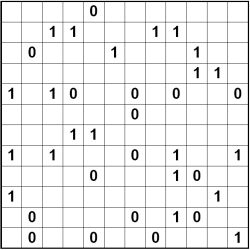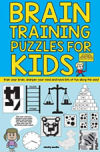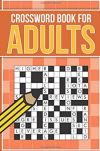Sun, 29 Nov 2020

Binary puzzles, also known as binaire puzzels in some European countries, are played on a rectangular or square grid. At the start of the puzzle, there are three possible states in which a cell can be: it can be empty, it can contain a zero, or it can contain a one. In the completed puzzle, there are only two valid states: either a cell contains a 1 or a 0. And hence the name of the puzzle: the Binary Puzzle.In a completed grid, there are certain rules that must be observed:

1. Repetition: no more than two cells in a row can contain the same number. Thus if there is a '1,1' then the cells either side must be '0'.

2. Equality: there must be an equal number of 0's and 1's in each row and column of the puzzle. Therefore there are the same number of 0's and 1's in the finished grid.

There is also a popular variant on the basic binary puzzle, in which each row and column must be unique, thus if the row '101010' appeared in a 6 x 6 puzzle then no other row or column could be identical.

As with most logic puzzles, there is only one solution, and that solution can be reached without any guesswork at all, but rather by simply applying basic logical rules that follow on from the rules of the game.

The simplest rule is to look for occasions where the same number occurs adjacent to itself in two cells, because then you know that the cells around that double occurrence must be the other number.

There is also a counting rule: if you know that in an 8x8 puzzle that there are 4 occurrences each of the zero and the one in a region then you can use this information to reduce the possible options for that row or column based on the numbers that are already placed (the givens) in that region of the binary puzzle.

Puzzles can be created in a range of difficulty levels, and in addition in a large range of different grid sizes too. Binary puzzles are usually supplied in PDF format, complete with solution. If you wish to buy binary puzzles (binaire puzzels) for publication, please do get in touch.

Noughts and Crosses Variant

A popular variant of the binary puzzle is called 'noughts and crosses', and replaces the 0s and 1s of the binary puzzle with noughts (0s) and crosses (Xs). The rules are otherwise exactly the same as the binary puzzle.

If you'd like to have a go at a puzzle, you can play a noughts and crosses puzzle online with our online player tool.

What to do next...

Individuals - You can play a large range of logic and number puzzles online at the Puzzle Connoisseur's Club

If you prefer to print puzzles, then take a look at Binary Puzzles Magazine

Here is a video explaining the rules of noughts and crosses puzzles:

Puzzle Solutions
i Newspaper Solutions

Book Ideas: For ChildrenBrain Training for KidsView a single page overview
list of puzzles

list of kids puzzles

Word Puzzles
Children's Puzzles
Wordsearch
CodeWords
Arrow words
Kriss Kross
Word Wheel

Number & Colouring Puzzles
Children's Sudoku
Samurai Sudoku
Jigsaw Sudoku
Futoshiki
Mind Games
Killer Sudoku
Kakuro
Chess Puzzles
A - Z of Puzzles
Colour By Number
Dot To Dot

Puzzle Tools
Puzzle FAQ
Codeword Solver
Rhyming Dictionary
Puzzle Solving Tools

Services
Puzzles for Apps
Article Writing
Themed Puzzle Pages